# Tennis racket theorem facts for kids

Kids Encyclopedia Facts

The tennis racket theorem or intermediate axis theorem is a result in classical mechanics describing the movement of a rigid body with three distinct principal moments of inertia. It is also dubbed the Dzhanibekov effect, after Russian cosmonaut Vladimir Dzhanibekov who noticed one of the theorem's logical consequences while in space in 1985 although the effect was already known for at least 150 years before that.

The theorem describes the following effect: rotation of an object around its first and third principal axes is stable, while rotation around its second principal axis (or intermediate axis) is not.

This can be demonstrated with the following experiment: hold a tennis racket at its handle, with its face being horizontal, and try to throw it in the air so that it will perform a full rotation around the horizontal axis perpendicular to the handle, and try to catch the handle. In almost all cases, during that rotation the face will also have completed a half rotation, so that the other face is now up. By contrast, it is easy to throw the racket so that it will rotate around the handle axis (the third principal axis) without accompanying half-rotation around another axis; it is also possible to make it rotate around the vertical axis perpendicular to the handle (the first principal axis) without any accompanying half-rotation.

The experiment can be performed with any object that has three different moments of inertia, for instance with a book, remote control or smartphone. The effect occurs whenever the axis of rotation differs only slightly from the object's second principal axis; air resistance or gravity are not necessary.

## Theory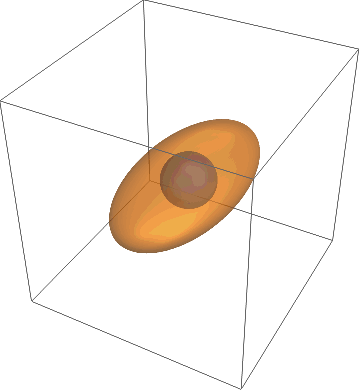A visualization of the instability of the intermediate axis. The magnitude of the angular momentum and the kinetic energy of a spinning object are both conserved. As a result, the angular velocity vector remains on the intersection of two ellipsoids.

The tennis racket theorem can be qualitatively analysed with the help of Euler's equations. Under torque–free conditions, they take the following form:\begin{align} I_1\dot{\omega}_1 &=(I_2-I_3)\omega_2\omega_3~~~~~~~~~~~~~~~~~~~~\text{(1)}\\ I_2\dot{\omega}_2 &=(I_3-I_1)\omega_3\omega_1~~~~~~~~~~~~~~~~~~~~\text{(2)}\\ I_3\dot{\omega}_3 &=(I_1-I_2)\omega_1\omega_2~~~~~~~~~~~~~~~~~~~~\text{(3)} \end{align}

Here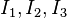$I_1, I_2, I_3$ denote the object's principal moments of inertia, and we assume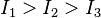$I_1 > I_2 > I_3$. The angular velocities around the object's three principal axes are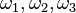$\omega_1, \omega_2, \omega_3$ and their time derivatives are denoted by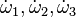$\dot\omega_1, \dot\omega_2, \dot\omega_3$.

### Stable rotation around the first and third principal axis

Consider the situation when the object is rotating around axis with moment of inertia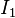$I_1$. To determine the nature of equilibrium, assume small initial angular velocities along the other two axes. As a result, according to equation (1),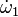$~\dot{\omega}_1$ is very small. Therefore, the time dependence of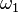$~\omega_1$ may be neglected.

Now, differentiating equation (2) and substituting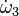$\dot{\omega}_3$ from equation (3),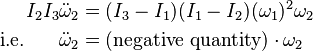\begin{align} I_2 I_3 \ddot{\omega}_2 &= (I_3-I_1) (I_1-I_2)(\omega_1)^2\omega_2 \\ \text{i.e. }~~~~ \ddot{\omega}_2 &= \text{(negative quantity)} \cdot \omega_2 \end{align}

because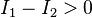$I_1-I_2 > 0$ and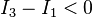$I_3-I_1 < 0$.

Note that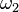$\omega_2$ is being opposed and so rotation around this axis is stable for the object.

Similar reasoning gives that rotation around axis with moment of inertia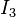$I_3$ is also stable.

### Unstable rotation around the second principal axis

Now apply the same analysis to axis with moment of inertia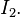$I_2.$ This time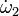$\dot{\omega}_{2}$ is very small. Therefore, the time dependence of$~\omega_2$ may be neglected.

Now, differentiating equation (1) and substituting$\dot{\omega}_3$ from equation (3),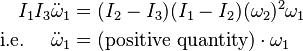\begin{align} I_1 I_3 \ddot{\omega}_1 &= (I_2-I_3) (I_1-I_2) (\omega_2)^2\omega_1\\ \text{i.e.}~~~~ \ddot{\omega}_1 &= \text{(positive quantity)} \cdot \omega_1 \end{align}

Note that$\omega_1$ is not opposed (and therefore will grow) and so rotation around the second axis is unstable. Therefore, even a small disturbance along other axes causes the object to 'flip'.Tennis racket theorem Facts for Kids. Kiddle Encyclopedia.